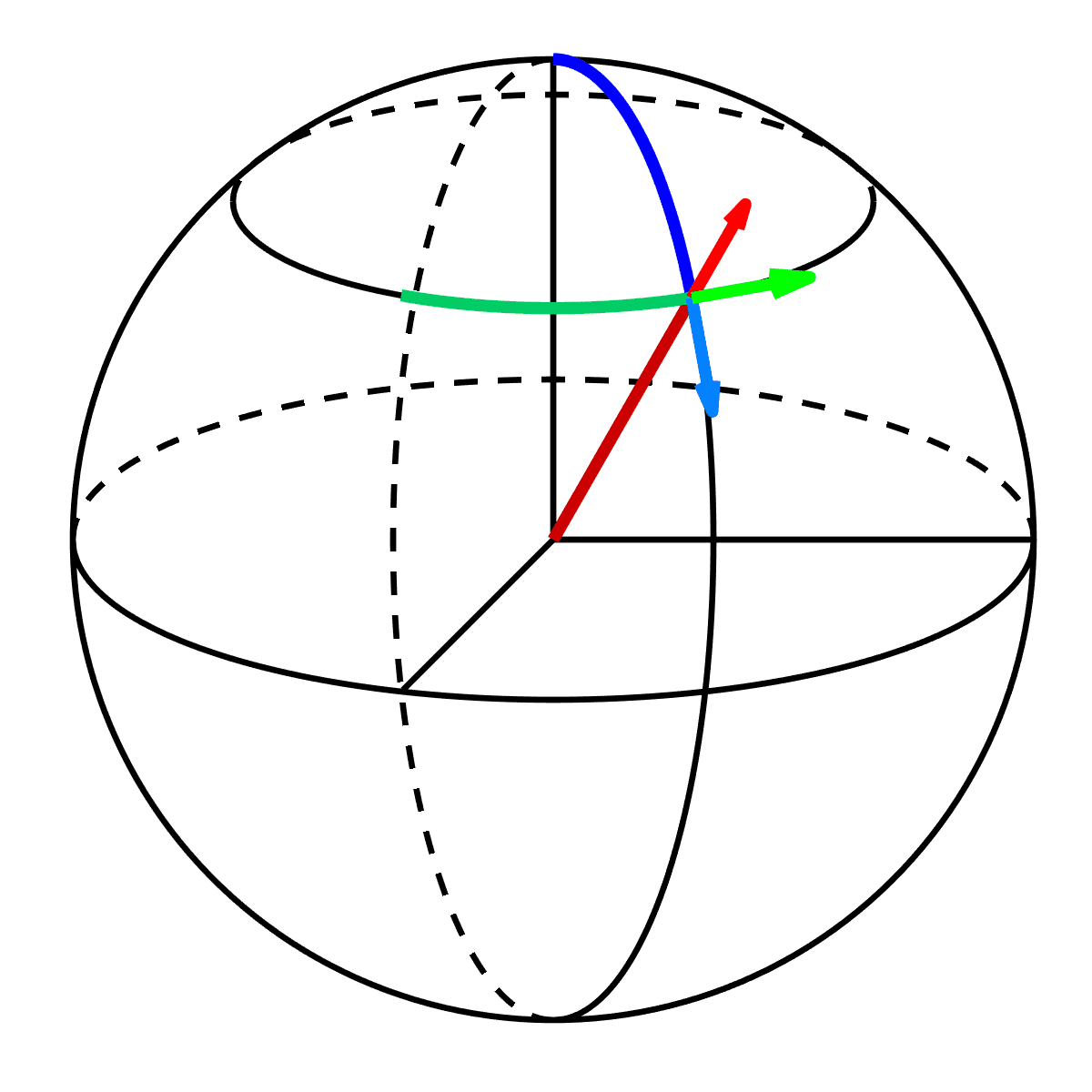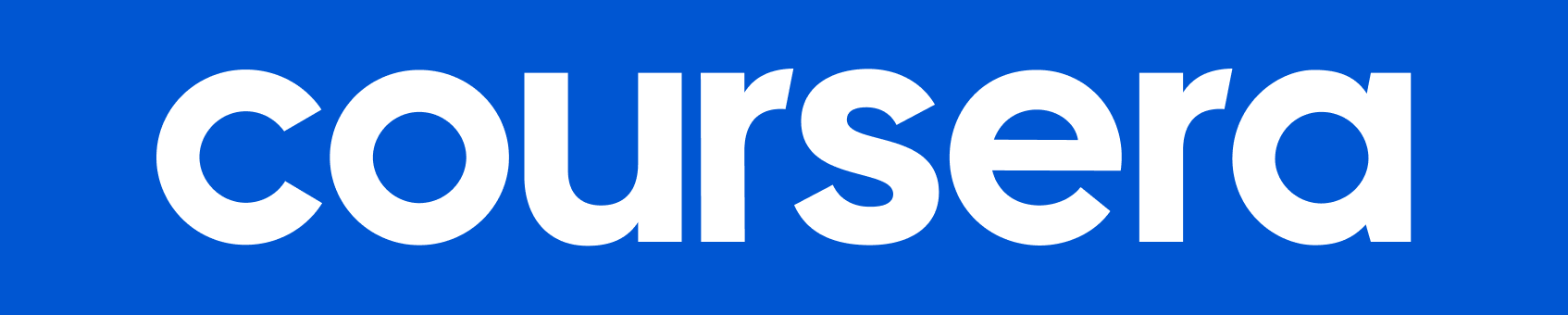\$49

# Vector Calculus for Engineers

Created by -

Jeffrey R. Chasnov TOP INSTRUCTOR
,
The Hong Kong University of Science and Technology

0.00

(0 ratings)

English

Wishlist

Overview

Watch the promotional video: https://youtu.be/qUseabHb6Vk Vector Calculus for Engineers covers both basic theory and applications. In the first week we learn about scalar and vector fields, in the second week about differentiating fields, in the third week about multidimensional integration and curvilinear coordinate systems. The fourth week covers line and surface integrals, and the fifth week covers the fundamental theorems of vector calculus, including the gradient theorem, the divergence theorem and Stokes’ theorem. These theorems are needed in core engineering subjects such as Electromagnetism and Fluid Mechanics. Instead of Vector Calculus, some universities might call this course Multivariable or Multivariate Calculus or Calculus 3. Two semesters of single variable calculus (differentiation and integration) are a prerequisite. The course is organized into 53 short lecture videos, with a few problems to solve following each video. And after each substantial topic, there is a short practice quiz. Solutions to the problems and practice quizzes can be found in instructor-provided lecture notes. There are a total of five weeks to the course, and at the end of each week there is an assessed quiz. Lecture notes can be downloaded from http://www.math.ust.hk/~machas/vector-calculus-for-engineers.pdfUSD 49Type: Online

This course includes

• Approx. 22 hours to complete
• Earn a Certificate upon completion
• Start instantly and learn at your own schedule.

Taken this course?

Share your experience with other students

ShareUSD 49Type: Online

This course includes

• Approx. 22 hours to complete
• Earn a Certificate upon completion
• Start instantly and learn at your own schedule.

Taken this course?

Share your experience with other students

Share

Vector Calculus for Engineers

Created by -

Jeffrey R. Chasnov TOP INSTRUCTOR
,
The Hong Kong University of Science and Technology

0.00

(0 ratings)

All Levels

Start Date: February 10th 2021

Course Description

Watch the promotional video: https://youtu.be/qUseabHb6Vk Vector Calculus for Engineers covers both basic theory and applications. In the first week we learn about scalar and vector fields, in the second week about differentiating fields, in the third week about multidimensional integration and curvilinear coordinate systems. The fourth week covers line and surface integrals, and the fifth week covers the fundamental theorems of vector calculus, including the gradient theorem, the divergence theorem and Stokes’ theorem. These theorems are needed in core engineering subjects such as Electromagnetism and Fluid Mechanics. Instead of Vector Calculus, some universities might call this course Multivariable or Multivariate Calculus or Calculus 3. Two semesters of single variable calculus (differentiation and integration) are a prerequisite. The course is organized into 53 short lecture videos, with a few problems to solve following each video. And after each substantial topic, there is a short practice quiz. Solutions to the problems and practice quizzes can be found in instructor-provided lecture notes. There are a total of five weeks to the course, and at the end of each week there is an assessed quiz. Lecture notes can be downloaded from http://www.math.ust.hk/~machas/vector-calculus-for-engineers.pdf

The information used on this page is how each course is described on the Coursera platform.

Course Structure

Tags

Mark CompleteJeffrey R. Chasnov TOP INSTRUCTOR,The Hong Kong University of Science and Technology

No Reviews at this moment.

#### Explore SkillqoreLearning

My Account

Blog

Featured Courses

RecommendedSkillqore

Universities

Institutes